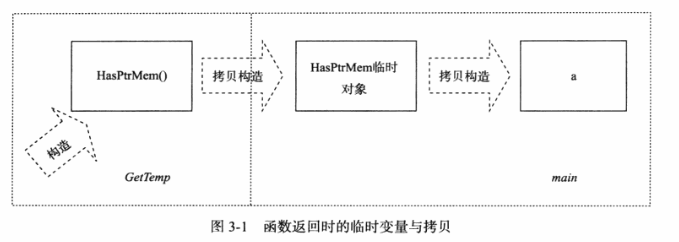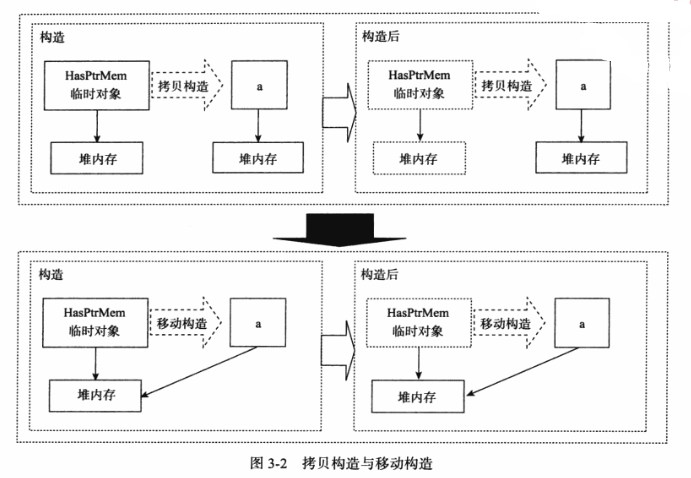# 通用为本，专用为末

## 【深入理解C++11】第三章

Posted by LudoArt on October 8, 2020

# 通用为本，专用为末

## 1. 继承构造函数

• 适用场景：当基类A有很多构造函数的版本，而派生类B想要拥有A那么多的构造方法时，会造成不便。
• 使用方法：通过using声明来声明继承基类的构造函数。
• 优点：书写便捷；节省目标代码空间（继承构造函数如果不被相关代码使用，编译器不会为其产生真正的函数代码）
• 缺点：只会初始化基类中的成员变量，对于派生类中的成员变量，则无能为力。

``````struct A
{
A(int i) {}
A(double d, int i) {}
A(float f, int i, const char* c) {}
// ...
};

struct B : A
{
using A::A;		// 继承构造函数
// ...
};

// 可以使用成员变量初始化的方式解决继承构造函数无法初始化派生类成员的问题
struct C : A
{
using A::A;		// 继承构造函数
int d {0};
};

int main()
{
C c{356};		// c.d被初始化为0
}
``````

​ 基类构造函数的参数会有默认值，对于继承构造函数来讲，参数的默认值是不会被继承的。

​ 事实上，默认值会导致基类产生多个构造函数的版本，这些函数版本都会被派生类继承。

• `A(int = 3, double = 2.4);`这是使用两个参数的情况
• `A(int = 3);`这是减掉一个参数的情况
• `A(const A &);`这是默认的复制构造函数
• `A();`这是不使用参数的情况

• `B(int, double);`这是一个继承构造函数
• `B(int);`这是减掉一个参数的继承构造函数
• `B(const B &);`这是复制构造函数，不是继承来的
• `B();`这是不包含参数的默认构造函数

​ 继承构造函数“冲突”的情况，通常发生在派生类拥有多个基类的时候。多个基类中的部分构造函数可能导致派生类中的继承构造函数的函数名、参数都相同，那么继承类中的冲突的继承构造函数将导致不合法的派生类代码。

``````struct A { A(int) {} };
struct B { B(int) {} };

struct C: A, B
{
// A和B的构造函数会导致C中重复定义相同类型的继承构造函数
using A::A;
using B::B;
// 可以通过显示定义继承类的冲突和构造函数，阻止隐式生成相应的继承构造函数来解决冲突
C(int) {}	// 这样就可以很好解决冲突问题了
};
``````

## 2. 委派构造函数

• 适用场景：多个构造函数基本相似，代码存在着很多重复。
• 使用方法：在初始化列表中调用“基准版本”的构造函数，即委派构造函数是在构造函数的初始化列表位置进行构造的、委派的。
• 优点：多构造函数的类编写将更加容易。
• 缺点：构造函数不能同时“委派”和使用初始化列表

``````class Info
{
public:
Info() { InitRest(); }
Info(int i) : Info() { type = i; }
Info(char e) : Info() { name = e; }

private:
void InitRest() { /* 其他初始化 */ }
int type {1};
char name { 'a' };
}
``````

`Info(int i) : Info(), type(i) {}` //无法通过编译

``````class Info
{
public:
Info() : Info(1, 'a') { }
Info(int i) : Info(i, 'a') { }
Info(char e) : Info(1, e) { }

private:
Info(int i, char e) : type(i), name(e) { /* 其他初始化 */ }
int type;
char name;
}
``````

``````struct Rule
{
int i, c;
Rule() : Rule(2) { }
Rule(int i) : Rule('c') { }
Rule(char c) : Rule(2) { }
};
``````

`Rule()``Rule(int)``Rule(char)`都依赖于别的构造函数，形成环委托构造关系，这样的代码通常会导致编译错误。

``````#include <list>
#include <vector>
#include <deque>
using namespace std;

class TDConstructed
{
template<class T> TDConstructed(T first, T last) :
l(first, last) {}
list<int> l;

public:
TDConstructed(vector<short> & v) :
TDConstructed(v.begin(), v.end()) {} // 委派构造函数
TDConstructed(deque<int> & d) :
TDConstructed(d.begin(), d.end()) {} // 委派构造函数
};
``````

``````#include <iostream>
using namespace std;

class DCExcept
{
public:
DCExcept(double d)
try : DCExcept(1, d) {
cout << "Run the body." << endl;
// 其他初始化
}
catch(...) {
cout << "caught exception." << endl;
}
private:
DCExcept(int i, double d){
cout << "going to throw!" << endl;
throw 0;
}
int type;
double data;
};

int main()
{
DCExcept a(1.2);
}
``````

going to throw!

caught exception.

## 3. 右值引用：移动语义和完美转发

### 3.1 指针成员与拷贝构造

``````#include <iostream>
using namespace std;

class HasPtrMem
{
public:
HasPtrMem(): d(new int(0)) {}
HasPtrMem(const HasPtrMem & h):
d(new int(*h.d)) {} // 拷贝构造函数，从堆中分配内存，并用*h.d初始化
~HasPtrMem() { delete d; }
int * d;
};

int main()
{
HasPtrMem a;
HasPtrMem b(a);	// 调用HasPtrMem的拷贝构造函数
cout << *a.d << endl;	// 0
cout << *b.d << endl;	// 0
} // 正常析构
``````

Q：这段代码和上一段代码的区别在于一个`const`关键字，为什么结果上会有这么大的不同？代码是否有错？

A：经实验得出以下结论：

1. `const`关键字加与不加区别不大，一般来说拷贝构造函数不需要修改拷贝对象的值，所以会加`const`关键字。

2. 结果没有不同，`a.d``b.d`指向了不同的内存地址，能够正常析构。

3. 第一个示例代码应该是错的，应该是不写拷贝构造函数的版本。不写拷贝构造函数的话，编译器会隐式生成一个，其作用是执行类似于`memcpy`的按位拷贝，这个时候就不能正常析构了，编译器会直接报错，因为`a.d``b.d`指向了同一块堆内存。

``````#include <iostream>
using namespace std;

class HasPtrMem
{
public:
HasPtrMem(): d(new int(0)) {}
HasPtrMem(HasPtrMem & h):
d(new int(*h.d)) {} // 拷贝构造函数，从堆中分配内存，并用*h.d初始化
~HasPtrMem() { delete d; }
int * d;
};

int main()
{
HasPtrMem a;
HasPtrMem b(a);	// 调用HasPtrMem的拷贝构造函数
cout << *a.d << endl;	// 0
cout << *b.d << endl;	// 0
} // 正常析构
``````

### 3.2 移动语义

``````#include <iostream>
using namespace std;

class HasPtrMem
{
HasPtrMem(): d(new int(0))
{
cout << "Construct: " << ++n_cstr << endl;
}
HasPtrMem(const HasPtrMem & h): d(new int(*h.d))
{
cout << "Copy construct: " << ++n_cptr << endl;
}
~HasPtrMem()
{
delete d; // 书上漏了这行，可能造成内存泄露
cout << "Destruct: " << ++n_dstr << endl;
}
int * d;
static int n_cstr;
static int n_dstr;
static int n_cptr;
};

int HasPtrMem::n_cstr = 0;
int HasPtrMem::n_dstr = 0;
int HasPtrMem::n_cptr = 0;

HasPtrMem GetTemp() { return HasPtrMem(); }

int main()
{
HasPtrMem a = GetTemp();
// 输出：
// Construct: 1
// Copy construct: 1
// Destruct: 1
// Copy construct: 2
// Destruct: 2
// Destruct: 3
}
````````````#include <iostream>
using namespace std;

class HasPtrMem
{
HasPtrMem(): d(new int(0))
{
cout << "Construct: " << ++n_cstr << endl;
}
HasPtrMem(const HasPtrMem & h): d(new int(*h.d))
{
cout << "Copy construct: " << ++n_cptr << endl;
}
HasPtrMem(HasPtrMem && h): d(h.d) // 移动构造函数
{
h.d = nullptr;	// 将临时值的指针成员置空
cout << "Move construct: " << ++n_mvtr << endl;
}
~HasPtrMem()
{
delete d;
cout << "Destruct: " << ++n_dstr << endl;
}
int * d;
static int n_cstr;
static int n_dstr;
static int n_cptr;
static int n_mvtr;
};

int HasPtrMem::n_cstr = 0;
int HasPtrMem::n_dstr = 0;
int HasPtrMem::n_cptr = 0;
int HasPtrMem::n_mvtr = 0;

HasPtrMem GetTemp()
{
HasPtrMem h;
cout << "Resource from " << __func__ << ": " << hex << h.d << endl;
return h;
}

int main()
{
HasPtrMem a = GetTemp();
cout << "Resource from " << __func__ << ": " << hex << a.d << endl;
// 输出：
// Construct: 1
// Resource from GetTemp: 0x603010
// Move construct: 1
// Destruct: 1
// Move construct: 2
// Destruct: 2
// Resource from main: 0x603010
// Destruct: 3
}
``````### 3.3 左值、右值与右值引用

``````#include <iostream>
using namespace std;

struct Copyable
{
Copyable() {}
Copyable(const Copyable &o)
{
cout << "Copied" << endl;
}
};

Copyable ReturnRvalue() { return Copyable(); }
void AcceptVal(Copyable) {}
void AcceptRef(const Copyable & ) {}

int main()
{
cout << "Pass by value: " << endl;
AcceptVal(ReturnRvalue());	// 临时值被拷贝传入
cout << "Pass by reference: " << endl;
AcceptRef(ReturnRvalue());	// 临时值被作为引用传递
}

// 输出：
// Pass by value:
// Copied
// Copied
// Pass by reference:
// Copied
``````

C++11中引用类型及其可以引用的值类型

### 3.4 std::move：强制转化为右值

`std::move`的唯一功能是将一个左值强制转化为右值引用，继而我们可以通过右值引用使用改值，以用于移动语义。从实现上讲，`std::move`基本等同于一个类型转换

`static_cast<T&&>(lvalue);`

``````#include <iostream>
using namespace std;

class HugeMem
{
public:
HugeMem(int size): sz(size > 0 ? size : 1) {
c = new int[sz];
}
~HugeMem() { delete [] c; }
HugeMem(HugeMem && hm): sz(hm.sz), c(hm.c) {
hm.c = nullptr;
}
int * c;
int sz;
};

class Moveable
{

Moveable(): i(new int(3)), h(1024) {}
~Moveable() { delete i; }
Moveable(Moveable && m): i(m.i), h(move(m.h)) {	// 强制转为右值，以调用移动构造函数
m.i = nullptr;
}
int * i;
HugeMem h;
};

Moveable GetTemp()
{
Moveable tmp = Moveable();
cout << hex << "Huge Mem from " << __func__
<< " @" << tmp.h.c << endl;	// Huge Mem from GetTemp @0x603030
return tmp;
}

int main()
{
Moveable a(GetTemp());
cout << hex << "Huge Mem from " << __func__
<< " @" << a.h.c << endl;	// Huge Mem from main @0x603030
}
``````

### 3.5 移动语义的一些其他问题

• `T Object(T &)`

• `T Object(const T &)`

• `T Object(T &&)`

``````template <class T>
void swap(T& a, T& b)
{
T tmp(move(a));
a = move(b);
b = move(tmp);
}
// 整个过程，代码都只会按照移动语义进行指针交换，不会有资源的释放与申请
``````

### 3.6 完美转发

C++11为了解决完美转发问题引入了“引用折叠”的新语言规则，并结合新的模板推导规则来完成完美转发。

C++11中的引用折叠规则
TR的类型定义 声明v的类型 v的实际类型
T& TR A&
T& TR& A&
T& TR&& A&
T&& TR A&&
T&& TR& A&
T&& TR&& A&&

``````#include <iostream>
using namespace std;

void RunCode(int && m) { cout << "rvalue ref" << endl; }
void RunCode(int & m) { cout << "lvalue ref" << endl; }
void RunCode(const int && m) { cout << "const rvalue ref" << endl; }
void RunCode(const int & m) { cout << "const lvalue ref" << endl; }

template <typename T> void PerfectForward(T &&t) { RunCode(forward<T>(t)); }

int main()
{
int a;
int b;
const int c = 1;
const int d = 0;

PerfectForward(a);	// lvalue ref
PerfectForward(move(b));	// rvalue ref
PerfectForward(c);	// const lvalue ref
PerfectForward(move(d));	// const rvalue ref
};
``````

``````#include <iostream>
using namespace std;

template <typename T, typename U> void PerfectForward(T &&t, U& Func)
{
cout << t << "\tforwarded..." << endl;
Func(forward<T>(t));
}

void RunCode(double && m) { }
void RunHome(double && h) { }
void RunComp(double && c) { }

int main()
{
PerfectForward(1.5, RunCode);	// 1.5	forwarded...
PerfectForward(8, RunHome);	// 8	forwarded...
PerfectForward(1.5, RunComp);	// 1.5	forwarded...
};
``````

## 4. 显示转换操作符

``````#include <iostream>
using namespace std;

struct Rational1
{
Rational1(int n = 0, int d = 1): num(n), den(d)
{
cout << __func__ << "(" << num << "/" << den << ")" << endl;
}
int num;	// 被除数
int den;	// 除数
};

struct Rational2
{
explicit Rational2(int n = 0, int d = 1): num(n), den(d) // explicit关键字修饰，不可被隐式调用
{
cout << __func__ << "(" << num << "/" << den << ")" << endl;
}
int num;	// 被除数
int den;	// 除数
};

void Display1(Rational1 ra)
{
cout << "Numberator: " << ra.num << " Denominator: " << ra.den << endl;
}

void Display2(Rational2 ra)
{
cout << "Numberator: " << ra.num << " Denominator: " << ra.den << endl;
}

int main()
{
Rational1 r1_1 = 11;	// Rational1(11/1)
Rational1 r1_2(12);	// Rational1(12/1)

Rational2 r2_1 = 21;	// 无法通过编译（因禁止被隐式构造）
Rational2 r2_2(22);		// Rational2(22/1)

Display1(1);		// Rational1(1/1)
// Numberator: 1 Denominator: 1
Display1(2);	// 无法通过编译（因禁止被隐式构造）
Display1(Rational2(2));	// Rational2(2/1)
// Numberator: 2 Denominator: 1
return 0;
}
``````

`explicit`关键字作用于类型转换操作符上，意味着只有在直接构造目标类型显示类型转换的时候可以使用该类型。但显示类型转换并没有完全禁止从源类型到目标类型的转换，不过由于此时拷贝构造和非显示类型转换不被允许，通常就不能通过赋值表达式或者函数参数的方式来产生这样一个目标类型。如下代码所示：

``````class ConvertTo {};
class Convertalbe
{
public:
explicit operator ConvertTo () const { return ConvertTo(); }
};
void Func(ConvertTo ct) {}
void test()
{
Convertalbe c;
ConvertTo ct(c);	// 直接初始化，通过
ConvertTo ct2 = c;	// 拷贝构造初始化，编译失败
ConvertTo ct3 = static_cast<ConvertTo>(c);	// 强制转化，通过
Func(c);	// 拷贝构造初始化，编译失败
}
``````

## 5. 列表初始化

### 5.1 初始化列表

• 等号“=”加上赋值表达式，比如 `int a = 3 + 4`
• 等号“=”加上花括号式的初始化列表，比如 `int a = { 3 + 4 }`
• 圆括号式的表达式列表，比如 `int a ( 3 + 4 )`
• 花括号式的初始化列表，比如 `int a { 3 + 4 }`

## 11. 一般化的SFINEA规则